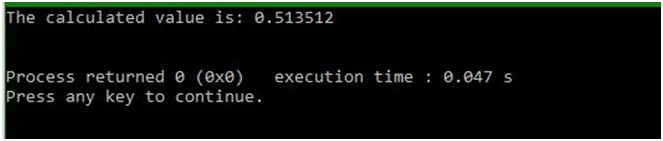# frexp() function of math.h in C

In this article, we are going to learn about the use frexp() function of math.h header file and then try to understand it with the help of an example.
Submitted by Manu Jemini, on March 30, 2018

This is an advance function used to get the absolute value of a floating point value in the form of mantissa which lies in the interval of [0.5, 1).

The Function takes two parameters, first is the number and second is the address of the integer.

Example:

```    Input:
X = 16.4324

Output:
Then the significant will be 0.513512
```

The Formula to find the mantissa is x = mantissa * 2 ^ exponent.

If the value of 'x' is zero, the function will return 0 and store it in the int.

The function also asks for the address of an integer which will get the normalizing fraction. This function is a part of math.h library and we must include it in the program.

## math.h - frexp() function Example in C

﻿

```#include <stdio.h>
#include <math.h>

int main()
{
float a,c;
// Defining variables
int b;

// Assigning value for getting frexp value
a = 16.4324;

// Calculating floating-point value into a fraction and an exponent
c = frexp(a, &b);

// Displaying the result for the user
printf("The calculated value is: %f \n\n", c);

return 0;
}
```

Output